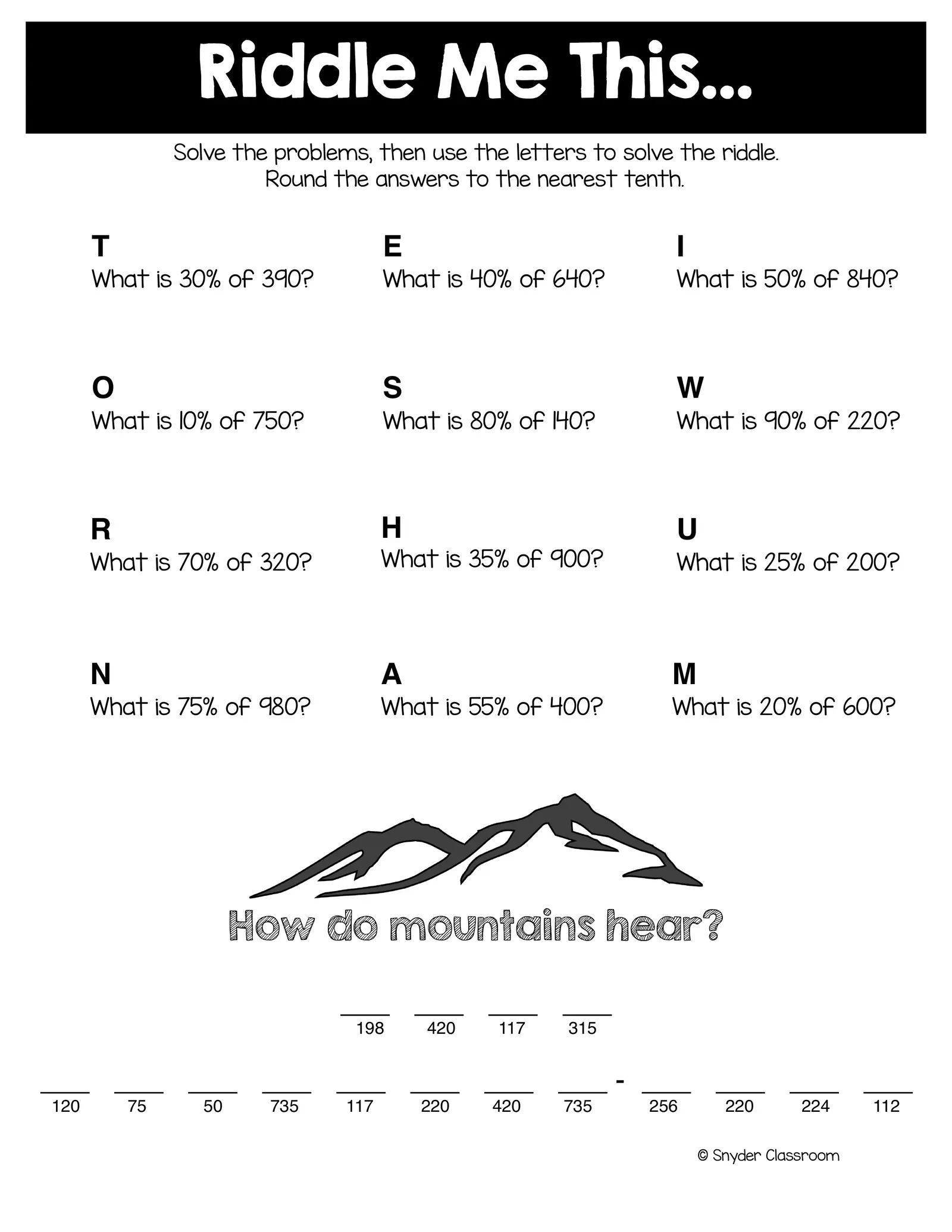# Part Percent Whole WS

Created
##### Pages

Riddle Me This… Solve the problems, then use the letters to solve the riddle. Round the answers to the nearest tenth. T E I What is 30% of 390? What is 40% of 640? What is 50% of 840? O S W What is 10% of 750? What is 80% of 140? What is 90% of 220? R H U What is 70% of 320? What is 35% of 900? What is 25% of 200? N A M What is 75% of 980? What is 55% of 400? What is 20% of 600? How do mountains hear? ___ ___ ___ ___ 198 420 117 315 ___ ___ ___ ___ ___ ___ ___ ___ - ___ ___ ___ ___ 120 75 50 735 117 220 420 735 256 220 224 112 © Snyder Classroom